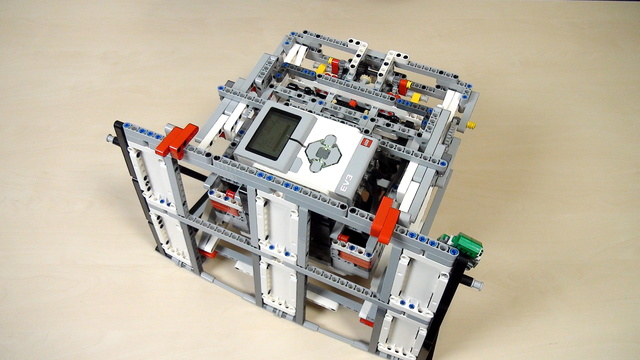# Improving FLL Robot Game. Our gear system calculation ProPreview

In the previous video, we found the correct answer for our task and it is 18.75, or is it?

• #471
• 08 Apr 2017
• 4:55

### Drawing the scheme of the system of gears

Redraw the system in the same way that we are doing it here.

### Calculate the ratios

Write the number of teeth on each of the gear wheels and calculate the ratios - the number of teeth on the driven wheel divided on the larger wheels on the driving wheel.

### Multiply all the ratios

After multiplying the ratios you will arrive at a number and this number is part of the answer to our question. "How do we calculate the number of rotation of the LEGO Mindstorms EV3 motor?"

### Robot Lifting Attachment With a scissors mechanism

For the FIRST LEGO League 2016-2017 Animal Allies we prepared an attachment for lifting the robot. The idea of the attachment is to show how you could lift the whole robot with a system of gear wheels, levers, and scissors constructions.

Built mainly from LEGO Mindstorms EV3 parts but could probably be constructed from NXT sets.

### English

The correct answer for our task is 18.75. This is the number of rotations that this small 12 teeth gear wheel connected to the driving axle must do to have the final wheel that's the driven wheel rotated by 90 degrees. 18.75. Let's see how we came up with this solution. Here is the process with a simple sheet of paper. First, we look at this 40 teeth gear wheel and this 8 teeth. And the system is a large wheel connected to a small wheel. Then this small gear wheel is on the same axle connected to another 24 teeth gear wheel which is again connected to a small gear wheel. And it's this one here. It is connected to a small gear wheel that's on the inside. I hope that you can see it. It's right here on the inside.

Right here. So, we have this. You can also see it in the instructions for this attachment. Then we have this small 8 teeth gear wheel connected to another one that's again a 24 teeth gear wheel connected to another one that's 8. And we have this 8 teeth gear wheel connected to this one - the back gear wheel that has 20 teeth. We have this one with 20 and then we finally have this one with 12. And the ratios are the following. I'll right them my side and I'll then rotate so that you can see it.

We have a system of gears where we have a 40 teeth gear wheel, an 8 teeth gear wheel. 24, 8, 24, 8, 20, 12. And the ratios are this one is 5, this one is 3, this one is 3 and this one is 1.6. This is how you do the calculation. And if you have more gear wheels in the system, you can continue with adding more wheels and more axles and calculating. Now, the final result is 5x3x3x1.6 which equals 75.

This result here 75 means that if the small driving wheel does 75 rotations, the final driven wheel will do 1 rotation. This one - 75, this one - 1 rotation. But we don't want this wheel to do 1 rotation we want it to do 1/4 of a rotation. We want to do 90 degrees with it. So, we must divide this result 75/4 and the result is 18.75.

Now, our mathematical model here gives us an answer that if we rotate this driving wheel to 18.75 rotations, the final driven wheel will rotate to 90 degrees. Let's test our mathematical model.

What happened? We did the calculation correctly. We found out that we have to do 18.75 rotations for 90 degrees with a large wheel but we're still not on the mission model. Our math is not wrong. Something else is happening here and we'll discuss this in the next videos.

## Courses and lessons with this Tutorial

This Tutorial is used in the following courses and lessons• 48
• 118:45
• 15• 8
• 0
• 2
• 3d_rotation 1